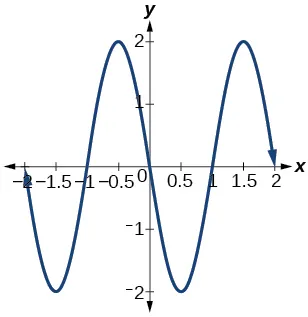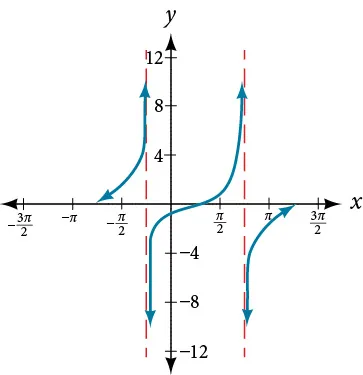Precalculus

# Practice Test

PrecalculusPractice Test

### Practice Test

For the following exercises, sketch the graph of each function for two full periods. Determine the amplitude, the period, and the equation for the midline.

1.

$f( x )=0.5sinx f( x )=0.5sinx$

2.

$f( x )=5cosx f( x )=5cosx$

3.

$f( x )=5sinx f( x )=5sinx$

4.

$f( x )=sin( 3x ) f( x )=sin( 3x )$

5.

$f( x )=−cos( x+ π 3 )+1 f( x )=−cos( x+ π 3 )+1$

6.

$f( x )=5sin( 3( x− π 6 ) )+4 f( x )=5sin( 3( x− π 6 ) )+4$

7.

$f( x )=3cos( 1 3 x− 5π 6 ) f( x )=3cos( 1 3 x− 5π 6 )$

8.

$f( x )=tan( 4x ) f( x )=tan( 4x )$

9.

$f( x )=−2tan( x− 7π 6 )+2 f( x )=−2tan( x− 7π 6 )+2$

10.

$f( x )=πcos( 3x+π ) f( x )=πcos( 3x+π )$

11.

$f( x )=5csc( 3x ) f( x )=5csc( 3x )$

12.

$f( x )=πsec( π 2 x ) f( x )=πsec( π 2 x )$

13.

$f( x )=2csc( x+ π 4 )−3 f( x )=2csc( x+ π 4 )−3$

For the following exercises, determine the amplitude, period, and midline of the graph, and then find a formula for the function.

14.

Give in terms of a sine function.15.

Give in terms of a sine function.16.

Give in terms of a tangent function.For the following exercises, find the amplitude, period, phase shift, and midline.

17.

$y=sin( π 6 x+π )−3 y=sin( π 6 x+π )−3$

18.

$y=8sin( 7π 6 x+ 7π 2 )+6 y=8sin( 7π 6 x+ 7π 2 )+6$

19.

The outside temperature over the course of a day can be modeled as a sinusoidal function. Suppose you know the temperature is 68°F at midnight and the high and low temperatures during the day are 80°F and 56°F, respectively. Assuming $t t$ is the number of hours since midnight, find a function for the temperature, $D, D,$ in terms of $t. t.$

20.

Water is pumped into a storage bin and empties according to a periodic rate. The depth of the water is 3 feet at its lowest at 2:00 a.m. and 71 feet at its highest, which occurs every 5 hours. Write a cosine function that models the depth of the water as a function of time, and then graph the function for one period.

For the following exercises, find the period and horizontal shift of each function.

21.

$g( x )=3tan( 6x+42 ) g( x )=3tan( 6x+42 )$

22.

$n( x )=4csc( 5π 3 x− 20π 3 ) n( x )=4csc( 5π 3 x− 20π 3 )$

23.

Write the equation for the graph in Figure 1 in terms of the secant function and give the period and phase shift.

Figure 1
24.

If $tanx=3, tanx=3,$ find $tan( −x ). tan( −x ).$

25.

If $secx=4, secx=4,$ find $sec( −x ). sec( −x ).$

For the following exercises, graph the functions on the specified window and answer the questions.

26.

Graph $m( x )=sin( 2x )+cos( 3x ) m( x )=sin( 2x )+cos( 3x )$ on the viewing window $[ −10,10 ] [ −10,10 ]$ by $[ −3,3 ]. [ −3,3 ].$ Approximate the graph’s period.

27.

Graph $n( x )=0.02sin( 50πx ) n( x )=0.02sin( 50πx )$ on the following domains in $x: x:$ $[ 0,1 ] [ 0,1 ]$ and $[ 0,3 ]. [ 0,3 ].$ Suppose this function models sound waves. Why would these views look so different?

28.

Graph $f( x )= sinx x f( x )= sinx x$ on $[ −0.5,0.5 ] [ −0.5,0.5 ]$ and explain any observations.

For the following exercises, let $f( x )= 3 5 cos( 6x ). f( x )= 3 5 cos( 6x ).$

29.

What is the largest possible value for $f( x )? f( x )?$

30.

What is the smallest possible value for $f( x )? f( x )?$

31.

Where is the function increasing on the interval $[ 0,2π ]? [ 0,2π ]?$

For the following exercises, find and graph one period of the periodic function with the given amplitude, period, and phase shift.

32.

Sine curve with amplitude 3, period $π 3 , π 3 ,$ and phase shift $( h,k )=( π 4 ,2 ) ( h,k )=( π 4 ,2 )$

33.

Cosine curve with amplitude 2, period $π 6 , π 6 ,$ and phase shift $( h,k )=( − π 4 ,3 ) ( h,k )=( − π 4 ,3 )$

For the following exercises, graph the function. Describe the graph and, wherever applicable, any periodic behavior, amplitude, asymptotes, or undefined points.

34.

$f( x )=5cos( 3x )+4sin( 2x ) f( x )=5cos( 3x )+4sin( 2x )$

35.

$f( x )= e sint f( x )= e sint$

For the following exercises, find the exact value.

36.

$sin −1 ( 3 2 ) sin −1 ( 3 2 )$

37.

$tan −1 ( 3 ) tan −1 ( 3 )$

38.

$cos −1 ( − 3 2 ) cos −1 ( − 3 2 )$

39.

$cos −1 ( sin( π ) ) cos −1 ( sin( π ) )$

40.

$cos −1 ( tan( 7π 4 ) ) cos −1 ( tan( 7π 4 ) )$

41.

$cos( sin −1 ( 1−2x ) ) cos( sin −1 ( 1−2x ) )$

42.

$cos −1 ( −0.4 ) cos −1 ( −0.4 )$

43.

$cos( tan −1 ( x 2 ) ) cos( tan −1 ( x 2 ) )$

For the following exercises, suppose $sint= x x+1 . sint= x x+1 .$ Evaluate the following expressions.

44.

$tant tant$

45.

$csct csct$

46.

Given Figure 2, find the measure of angle $θ θ$ to three decimal places. Answer in radians.

Figure 2

For the following exercises, determine whether the equation is true or false.

47.

$arcsin( sin( 5π 6 ) )= 5π 6 arcsin( sin( 5π 6 ) )= 5π 6$

48.

$arccos( cos( 5π 6 ) )= 5π 6 arccos( cos( 5π 6 ) )= 5π 6$

49.

The grade of a road is 7%. This means that for every horizontal distance of 100 feet on the road, the vertical rise is 7 feet. Find the angle the road makes with the horizontal in radians.

Order a print copy

As an Amazon Associate we earn from qualifying purchases.